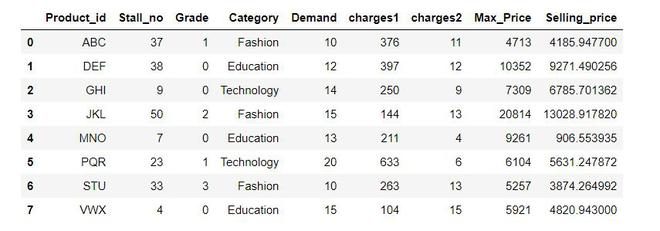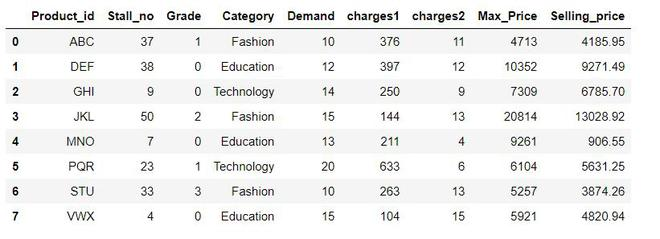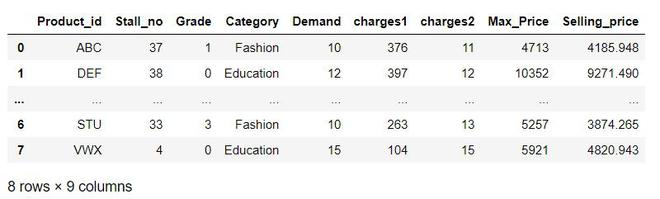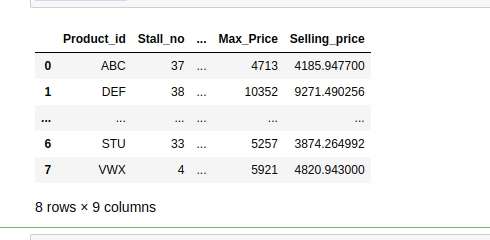Open in App
Not now

# How to Pretty Print an Entire Pandas Series or DataFrame?

• Last Updated : 14 Sep, 2022

In this article, we are going to see how to Pretty Print the entire pandas Series / Dataframe.  There are various pretty print options are available for use with this method. Here we will discuss 3 ways to Pretty Print the entire Pandas Dataframe:

## Python3

 `import` `pandas as pd` `# Create a dataframe``df ``=` `pd.DataFrame({``  ``'Product_id'``: [``'ABC'``, ``'DEF'``, ``'GHI'``, ``'JKL'``,``                 ``'MNO'``, ``'PQR'``, ``'STU'``, ``'VWX'``],``  ` `  ``'Stall_no'``: [``37``, ``38``, ``9``, ``50``, ``7``, ``23``, ``33``, ``4``],``  ``'Grade'``: [``1``, ``0``, ``0``, ``2``, ``0``, ``1``, ``3``, ``0``],``  ` `  ``'Category'``: [``'Fashion'``, ``'Education'``, ``'Technology'``,``               ``'Fashion'``, ``'Education'``, ``'Technology'``,``               ``'Fashion'``, ``'Education'``],``  ` `  ``'Demand'``: [``10``, ``12``, ``14``, ``15``, ``13``, ``20``, ``10``, ``15``],``  ``'charges1'``: [``376``, ``397``, ``250``, ``144``, ``211``, ``633``, ``263``, ``104``],``  ``'charges2'``: [``11``, ``12``, ``9``, ``13``, ``4``, ``6``, ``13``, ``15``],``  ``'Max_Price'``: [``4713``, ``10352``, ``7309``, ``20814``, ``9261``,``                ``6104``, ``5257``, ``5921``],``  ` `  ``'Selling_price'``: [``4185.9477``, ``9271.490256``, ``6785.701362``,``                    ``13028.91782``, ``906.553935``, ``5631.247872``,``                    ``3874.264992``, ``4820.943``]})``display(df)`

Output:Some Important terms to use in pretty print options are discussed below:

• display.max_columns: The maximum number of columns pandas should print. If None is provided as an argument all columns are printed.
• display.max_rows: The maximum number of rows pandas should print. If None is provided as an argument all rows are printed.
• display.precision: Floating point output precision in terms of a number of places after the decimal, for regular formatting as well as scientific notation.
• display.width: Width of the display in characters. If set to None, pandas will correctly auto-detect the width.

### Reduce the Size of a Pandas Dataframe using pd.set_options()

We will use some options of the set_options() method on the above df to see all rows, all columns, all columns in one row with center-aligned column headers, and rounding the number of places after the decimal for each floating value to 2.

## Python3

 `pd.set_option(``'display.max_rows'``, ``None``)``pd.set_option(``'display.max_columns'``, ``None``)``pd.set_option(``'display.width'``, ``1000``)``pd.set_option(``'display.colheader_justify'``, ``'center'``)``pd.set_option(``'display.precision'``, ``2``)``display(df)`

Output:

Once set through pd.set_options() method, the same settings are used with all the next Dataframe printing commands.### Reduce the Size of a Pandas Dataframe using pd.option_context()

The pd.set_option() method provides permanent setting for displaying dataframe. pd.option_context() temporarily sets the options in with statement context. Following code prints the above df with 4 rows, all columns, all columns in one row with left-aligned column headers, and rounding the number of places after the decimal for each floating value.

## Python3

 `with pd.option_context(``'display.max_rows'``, ``5``,``                       ``'display.max_columns'``, ``None``,``                       ``'display.width'``, ``1000``,``                       ``'display.precision'``, ``3``,``                       ``'display.colheader_justify'``, ``'left'``):``    ``display(df)`

Output:### Reduce the Size of a Pandas Dataframe using options.display

Following code prints the above df with 4 rows, 4 columns, all columns in one row with left-aligned column headers, and not rounding the number of places after the decimal for each floating value.

## Python3

 `import` `pandas as pd``import` `numpy as np` `def` `display_options():``    ` `    ``display ``=` `pd.options.display``    ``display.max_columns ``=` `5``    ``display.max_rows ``=` `4``    ``display.max_colwidth ``=` `222``    ``display.width ``=` `None``    ``return` `None` `display_options()``display(df)`

Output:My Personal Notes arrow_drop_up